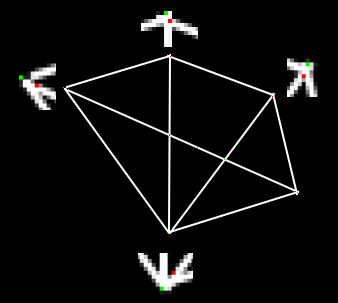# OpenCV-Python 系列 三十六 | 哈里斯角检测

### 目标

– 我们将了解”Harris Corner Detection”背后的概念。
– 我们将看到以下函数：cv.cornerHarris(),cv.cornerSubPix()

### 理论

E(u,v) = sum_{x,y} underbrace{w(x,y)}_text{window function} , [underbrace{I(x+u,y+v)}_text{shifted intensity}-underbrace{I(x,y)}_text{intensity}]^2

E(u,v) approx begin{bmatrix} u & v end{bmatrix} M begin{bmatrix} u v end{bmatrix}

M = sum_{x,y} w(x,y) begin{bmatrix}I_x I_x & I_x I_y I_x I_y & I_y I_y end{bmatrix}

R = det(M) – k(trace(M))^2

• det(M)=lambda_1lambda_2
• trace(M)=lambda_1+lambda_2
• lambda_1 and lambda_2M 的特征值

• |R|较小，这在lambda_1lambda_2较小时发生，该区域平坦。
• 当$R<0$时（当$lambda_1 >>lambda_2$时发生，反之亦然），该区域为边。
• R很大时，这发生在lambda_1lambda_2大且lambda_1~lambda_2时，该区域是角。### OpenCV中的哈里斯角检测

img – 输入图像，应为灰度和float32类型。
blockSize – 是拐角检测考虑的邻域大小
ksize – 使用的Sobel导数的光圈参数。
k – 等式中的哈里斯检测器自由参数。

import numpy as np
import cv2 as cv
filename = 'chessboard.png'
gray = cv.cvtColor(img,cv.COLOR_BGR2GRAY)
gray = np.float32(gray)
dst = cv.cornerHarris(gray,2,3,0.04)
#result用于标记角点，并不重要
dst = cv.dilate(dst,None)
#最佳值的阈值，它可能因图像而异。
img[dst>0.01*dst.max()]=[0,0,255]
cv.imshow('dst',img)
if cv.waitKey(0) & 0xff == 27:
cv.destroyAllWindows()### SubPixel精度的转角

import numpy as np
import cv2 as cv
filename = 'chessboard2.jpg'
gray = cv.cvtColor(img,cv.COLOR_BGR2GRAY)
# 寻找哈里斯角
gray = np.float32(gray)
dst = cv.cornerHarris(gray,2,3,0.04)
dst = cv.dilate(dst,None)
ret, dst = cv.threshold(dst,0.01*dst.max(),255,0)
dst = np.uint8(dst)
# 寻找质心
ret, labels, stats, centroids = cv.connectedComponentsWithStats(dst)
# 定义停止和完善拐角的条件
criteria = (cv.TERM_CRITERIA_EPS + cv.TERM_CRITERIA_MAX_ITER, 100, 0.001)
corners = cv.cornerSubPix(gray,np.float32(centroids),(5,5),(-1,-1),criteria)
# 绘制
res = np.hstack((centroids,corners))
res = np.int0(res)
img[res[:,1],res[:,0]]=[0,0,255]
img[res[:,3],res[:,2]] = [0,255,0]
cv.imwrite('subpixel5.png',img)